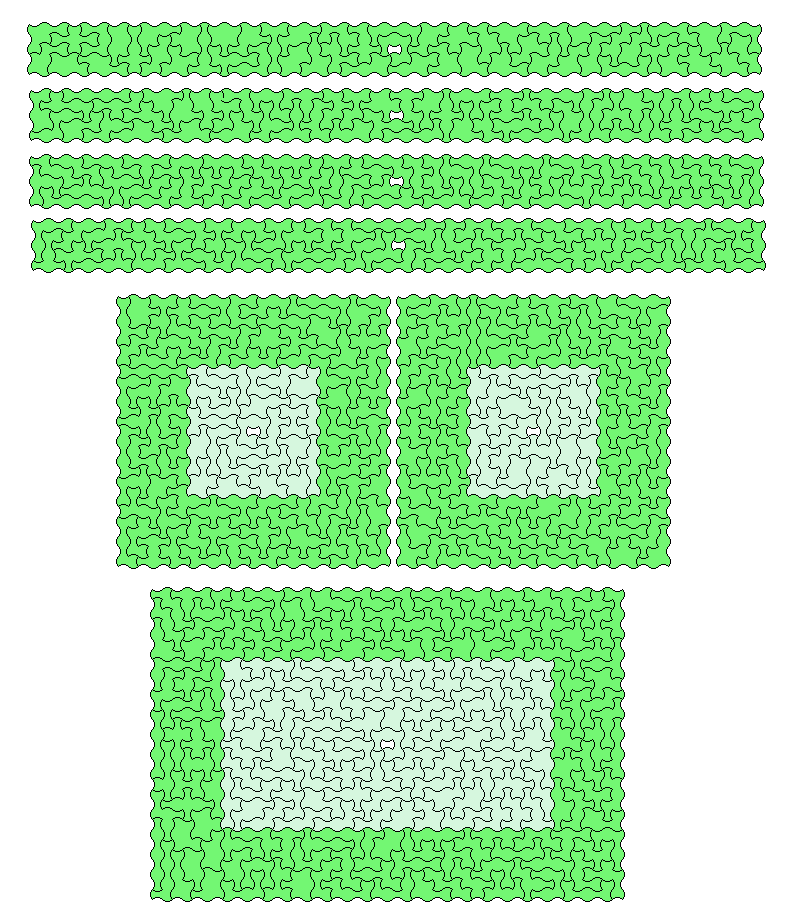## 7 Arc-Squares

208 two-sided pieces, total area = 1456.

Since there is a piece with a hole you can look for n congruent constructions with one hole each giving a total area of 1456+n.

n=4: four rectangles 5x73 each with a center hole
n=2: two squares 27x27 each with a center hole
n=1: one rectangle 31x47 with one holeindex